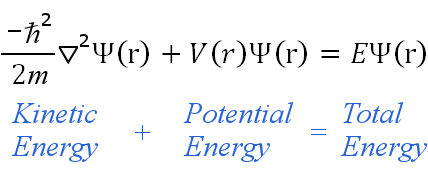# Schrodinger's Equation – A key equation in Quantum Mechanics

Until the beginning of the 20th century, we have developed laws and equations which govern the behaviour of relatively larger objects. In the early 20th century, we started digging deeper and deeper into the matter and found out that the laws we have developed over centuries don’t work at atomic and sub-atomic levels. This was the beginning of Quantum Physics.
Quantum Mechanics was started by Max Planck who is known as the Father of Quantum Mechanics. Quantum Mechanics tells that matter also have wave-particle duality and it is significant at the atomic scale. Thus it was thought that a wave equation might explain the properties and thus the first such wave equation was developed by Erwin Schrodinger and is named as Schrodinger’s equation.
Schrodinger’s wave equation looks like:is the reduced Planck constant,
m is the electron mass,is the Laplacian operator,
Ψ is the wave function,
V is the potential energy,
is the energy eigenvalue,
(r) denotes the quantities are functions of spherical polar coordinates (r, θ, φ)
As always, I am not going to bore you explaining those complex terms. Instead, let us know why is this equation so important. We all know about Newton’s laws of motion, they changed the course of physics as we could tell the destination of a moving body by knowing its initial conditions. Schrodinger’s role in Quantum mechanics is somewhat similar to that of Newton.
The Schrodinger equation is used to find the allowed energy levels of quantum mechanical systems (such as atoms, or transistors). The associated wavefunction gives the probability of finding the particle at a certain position. A particle whose position and momentum was completely unpredictable according to the uncertainty principle got at least boundaries by using Schrodinger’s equation. It really means a lot. Though there are many implications of this equation, the above one is the most important.
I hope you got a glimpse of Schrodinger’s equation.